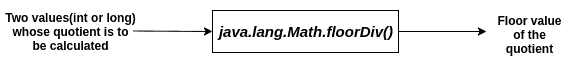# Java Math floorDiv() Function: Complete Guide

The floorDiv() method in Java gives the same result as the standard division, which operates under the “round to zero” rounding mode (truncation). However, for negative quotients, the results of normal division and that obtained by the floorDiv() method differ. This is because the floorDiv() method operates under the “round towards negative infinity” rounding mode. An example to differentiate between the two will be discussed in a later section.

## Java Math floorDiv()

Java Math floorDiv() is a built-in method that carries out the division between two ints (or long) values and returns the floor value of the quotient. The floor value of any real number is the nearest integral value less than the number. If the number is already an integral value, the floor value is the number itself.

### Syntax

```public static int floorDiv(int a, int b)
public static long floorDiv(long a, long b)```

### Parameter(s)

Two int(or long) values whose quotient is to be calculated.

### Return Value

The floor value of the quotient.

### Throws

ArithmeticException if the divisor is zero.

See the following figure.#### Note

1. If the dividend is Integer, MIN_VALUE, and the divisor is -1, an integer overflow occurs, and the method returns Integer.MIN_VALUE itself.
2. If the dividend is Long, MIN_VALUE, and the divisor is -1, an integer overflow occurs, and the method returns Long.MIN_VALUE itself.

Consider the following examples.

#### Example1.java: The following example demonstrates the use of this method.

```public class Example1 {
public static void main(String[] args) {
int a = 4;
int b = 3;

long c = 9;
long d = 6;

System.out.println(Math.floorDiv(a, b));
System.out.println(Math.floorDiv(c, d));
}
}
```

#### Output

```->javac Example1.java
->java Example1
1
1
```

#### Example2.java: The following example demonstrates the difference between the result obtained by normal division and that obtained by the floorDiv() method.

```public class Example2 {
public static void main(String[] args) {
int a = -4;
int b = 3;

System.out.println(a / b);
System.out.println(Math.floorDiv(a, b));
}
}
```

#### Output

```->javac Example2.java
->java Example2
-1
-2
```

#### Example3.java: The following example demonstrates the situation of a zero divisor.

```public class Example3 {
public static void main(String[] args) {
int a = 2;
int b = 0;

System.out.println(Math.floorDiv(a, b));
}
}
```

#### Output

```->javac Example3.java
->java Example3
Exception in thread "main" java.lang.ArithmeticException: / by zero
at java.lang.Math.floorDiv(Math.java:1052)
at Example3.main(Example3.java:6)```

#### Example4.java: The following example demonstrates the situation of dividend as Integer.MIN_VALUE and divisor as -1.

```public class Example4 {
public static void main(String[] args) {
int a = Integer.MIN_VALUE;
int b = -1;

System.out.println(a);
System.out.println(Math.floorDiv(a, b));
}
}
```

#### Output

```->javac Example4.java
->java Example4
-2147483648
-2147483648
```

#### Example5.java: The following example demonstrates the situation of dividend as Long.MIN_VALUE and divisor as -1.

```public class Example5 {
public static void main(String[] args) {
long a = Long.MIN_VALUE;
long b = -1;

System.out.println(a);
System.out.println(Math.floorDiv(a, b));
}
}
```

#### Output

```->javac Example5.java
->java Example5
-9223372036854775808
-9223372036854775808
```